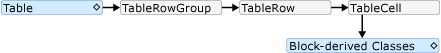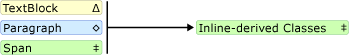# TextElement Content Model Overview

This content model overview describes the supported content for a TextElement. The Paragraph class is a type of TextElement. A content model describes what objects/elements can be contained in others. This overview summarizes the content model used for objects derived from TextElement. For more information, see Flow Document Overview.

## Content Model Diagram

The following diagram summarizes the content model for classes derived from TextElement as well as how other non- `TextElement` classes fit into this model.As can be seen from the preceding diagram, the children allowed for an element are not necessarily determined by whether a class is derived from the Block class or an Inline class. For example, a Span (an Inline-derived class) can only have Inline child elements, but a Figure (also an Inline-derived class) can only have Block child elements. Therefore, a diagram is useful for quickly determining what element can be contained in another. As an example, let's use the diagram to determine how to construct the flow content of a RichTextBox.

1. A RichTextBox must contain a FlowDocument which in turn must contain a Block-derived object. The following is the corresponding segment from the preceding diagram.Thus far, this is what the markup might look like.

``````<RichTextBox>
<FlowDocument>
<!-- One or more Block-derived object… -->
</FlowDocument>
</RichTextBox>
``````
2. According to the diagram, there are several Block elements to choose from including Paragraph, Section, Table, List, and BlockUIContainer (see Block-derived classes in the preceding diagram). Let's say we want a Table. According to the preceding diagram, a Table contains a TableRowGroup containing TableRow elements, which contain TableCell elements which contain a Block-derived object. The following is the corresponding segment for Table taken from the preceding diagram.The following is the corresponding markup.

``````<RichTextBox>
<FlowDocument>
<Table>
<TableRowGroup>
<TableRow>
<TableCell>
<!-- One or more Block-derived object… -->
</TableCell>
</TableRow>
</TableRowGroup>
</Table>
</FlowDocument>
</RichTextBox>
``````
3. Again, one or more Block elements are required underneath a TableCell. To make it simple, let's place some text inside the cell. We can do this using a Paragraph with a Run element. The following is the corresponding segments from the diagram showing that a Paragraph can take an Inline element and that a Run (an Inline element) can only take plain text.The following is the entire example in markup.

``````<Page xmlns="http://schemas.microsoft.com/winfx/2006/xaml/presentation"
xmlns:x="http://schemas.microsoft.com/winfx/2006/xaml">
<RichTextBox>
<FlowDocument>

<!-- Normally a table would have multiple rows and multiple
cells but this code is for demonstration purposes.-->
<Table>
<TableRowGroup>
<TableRow>
<TableCell>
<Paragraph>

<!-- The schema does not actually require
explicit use of the Run tag in markup. It
is only included here for clarity. -->
<Run>Paragraph in a Table Cell.</Run>
</Paragraph>
</TableCell>
</TableRow>
</TableRowGroup>
</Table>

</FlowDocument>
</RichTextBox>
</Page>
``````

## Working with TextElement Content Programmatically

The contents of a TextElement is made up by collections and so programmatically manipulating the contents of TextElement objects is done by working with these collections. There are three different collections used by TextElement -derived classes:

You can manipulate (add or remove items) from these collections using the respective properties of Inlines, Blocks, and ListItems. The following examples show how to manipulate the contents of a Span using the Inlines property.

Note

Table uses several collections to manipulate its contents, but they are not covered here. For more information, see Table Overview.

The following example creates a new Span object, and then uses the `Add` method to add two text runs as content children of the Span.

``````Span spanx = new Span();
spanx.Inlines.Add(new Run("A bit of text content..."));
spanx.Inlines.Add(new Run("A bit more text content..."));
``````
``````Dim spanx As New Span()
spanx.Inlines.Add(New Run("A bit of text content..."))
spanx.Inlines.Add(New Run("A bit more text content..."))
``````

The following example creates a new Run element and inserts it at the beginning of the Span.

``````Run runx = new Run("Text to insert...");
spanx.Inlines.InsertBefore(spanx.Inlines.FirstInline, runx);
``````
``````Dim runx As New Run("Text to insert...")
spanx.Inlines.InsertBefore(spanx.Inlines.FirstInline, runx)
``````

The following example deletes the last Inline element in the Span.

``````spanx.Inlines.Remove(spanx.Inlines.LastInline);
``````
``````spanx.Inlines.Remove(spanx.Inlines.LastInline)
``````

The following example clears all of the contents (Inline elements) from the Span.

``````spanx.Inlines.Clear();
``````
``````spanx.Inlines.Clear()
``````